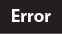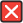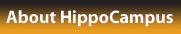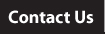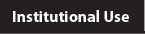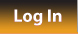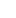More OER Help Center Log In About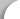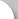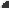PlaylistTOPICS
 Variable Expressions show details
 Simple Equations show details
 Equations 2 show details
 Equations 3 show details
 Algebra: Linear Equations 4 show details
 Algebra: Solving Inequalities show details
 Algebra: graphing lines 1 show details
 Algebra: Slope and Y-intercept intuition show details
 Algebra: Slope show details
 Algebra: Slope 2 show details
 Algebra: Slope 3 show details
 Systems of Equations show details
 Slope and Y-intercept Intuition show details
 The Coordinate Plane show details
 Graphing Using Intercepts show details
 Graphs of Linear Equations show details
 Slope and Rate of Change show details
 Direct Variation Models show details
 Linear Function Graphs show details
 Word Problem Solving 4 show details
 Linear Equations in Slope Intercept Form show details
 Linear Equations in Point Slope Form show details
 Equations of Parallel and Perpendicular Lines show details
 Using a Linear Model show details
 Patterns and Equations show details
 Equations and Inequalities show details
 Domain and Range of a Function show details
 Functions as Graphs show details
 Word Problem Solving Strategies show details
 Distributive Property show details
 One Step Equations show details
 Two-Step Equations show details
 Multi-Step Equations show details
 Equations with Variables on Both Sides show details
 Inequalities Using Addition and Subtraction show details
 Inequalities Using Multiplication and Division show details
 Compound Inequalities show details
 Multi-Step Inequalities show details
 Absolute Value Equations show details
 Absolute Value Inequalities show details
 Graphing Inequalities show details
 Solving Linear Systems by Graphing show details
 Solving Linear Systems by Substitution show details
 Solving Systems of Equations by Elimination show details
 Solving Systems of Equations by Multiplication show details
 Special Types of Linear Systems show details
 Level 1 multiplying expressions show details
 Solving a quadratic by factoring show details
 Introduction to the quadratic equation show details
 Quadratic Equation part 2 show details
 Completing the square show details
 Exponent Properties Involving Products show details
 Exponent Properties Involving Quotients show details
 Zero, Negative, and Fractional Exponents show details
 Pythagorean Theorem show details
 Pythagorean Theorem 3 show details
 Polynomial Division show details
 Factoring Special Products show details
 Factor by Grouping and Factoring Completely show details
 Graphs of Quadratic Functions show details
 Solving Quadratic Equations by Graphing show details
 Solving Quadratic Equations by Square Roots show details
 Solving Quadratic Equations by Completing the Square show details
 Using the Quadratic Formula show details
 Proof of Quadratic Formula show details
 Discriminant of Quadratic Equations show details
 Distance Formula show details
 Midpoint Formula show details
 Simplifying Rational Expressions show details
 Multiplying and Dividing Rational Expressions show details
 Adding Rational Expressions Example 1 show details
 Adding Rational Expressions Example 2 show details
 Adding Rational Expressions Example 3 show details
 Solving Rational Equations show details
 i and Imaginary numbers show details
 Complex Numbers (part 1) show details
 Complex Numbers (part 2) show details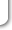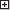maximize